Construction of line parallel to given line, through point not on line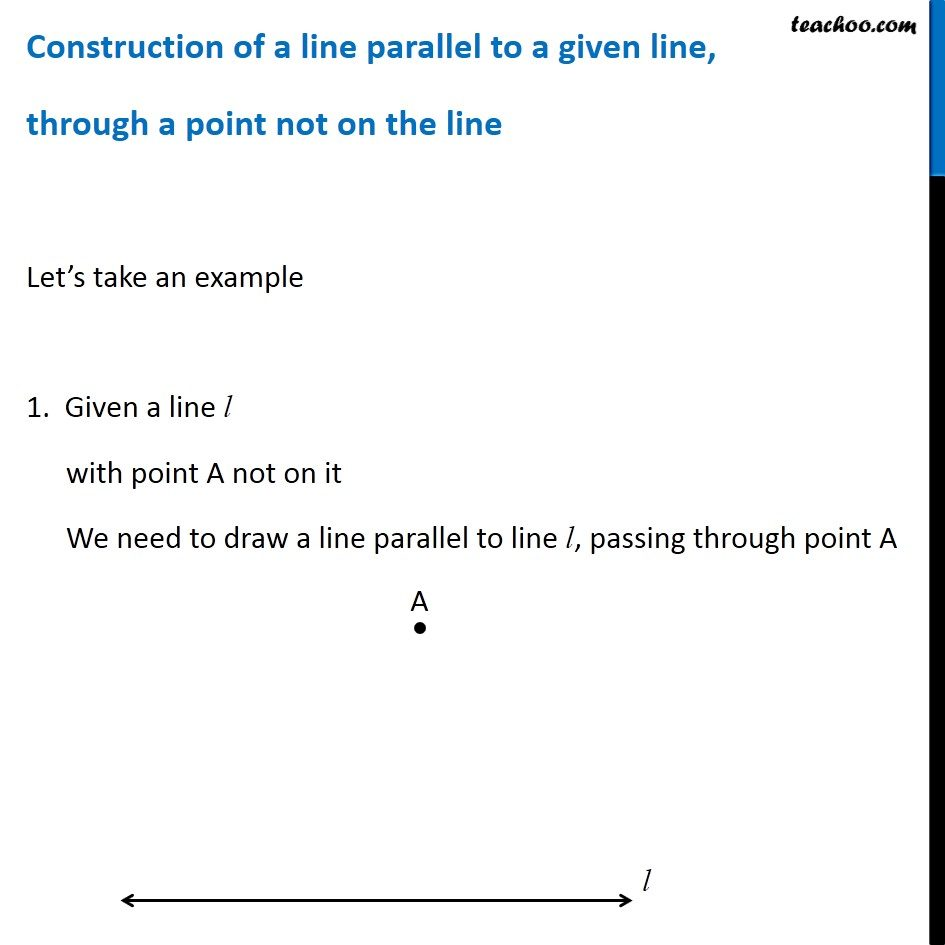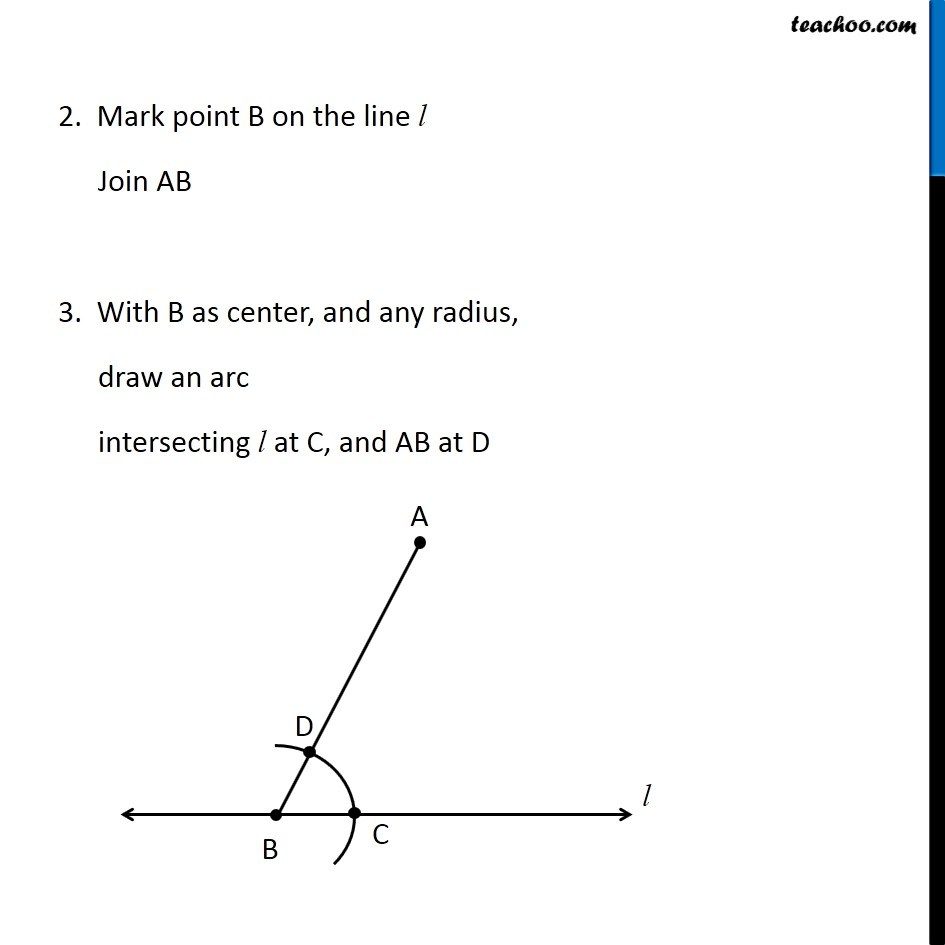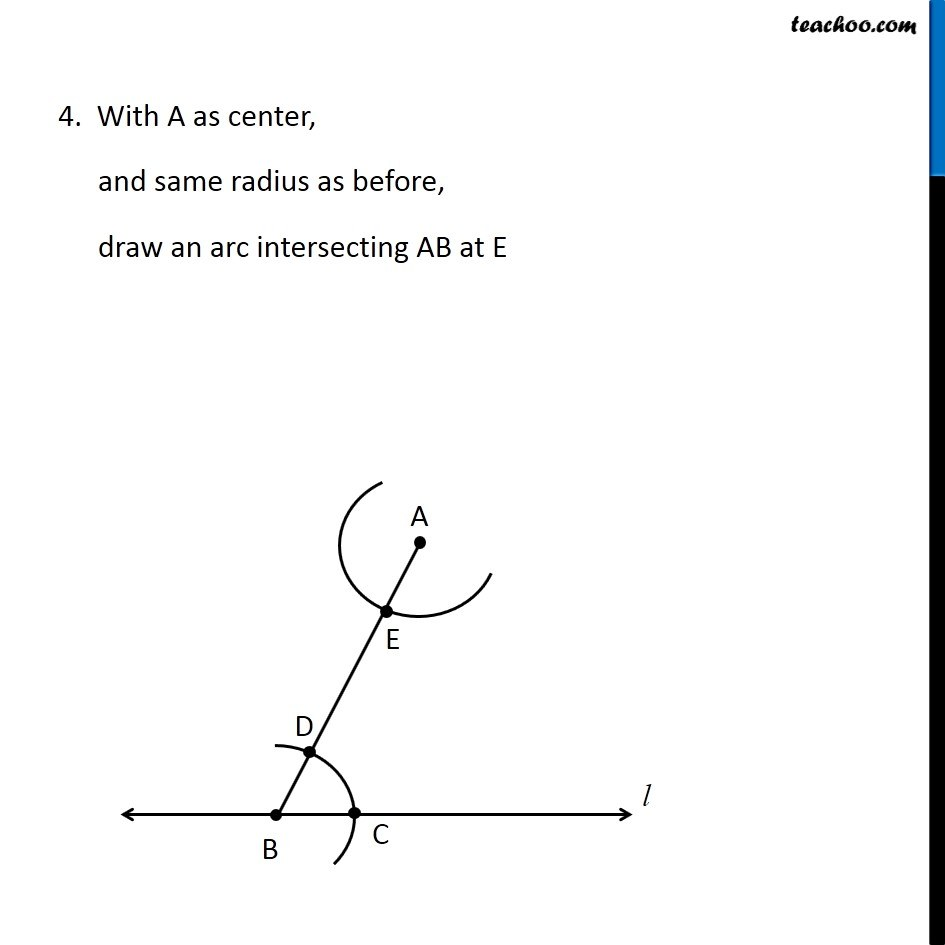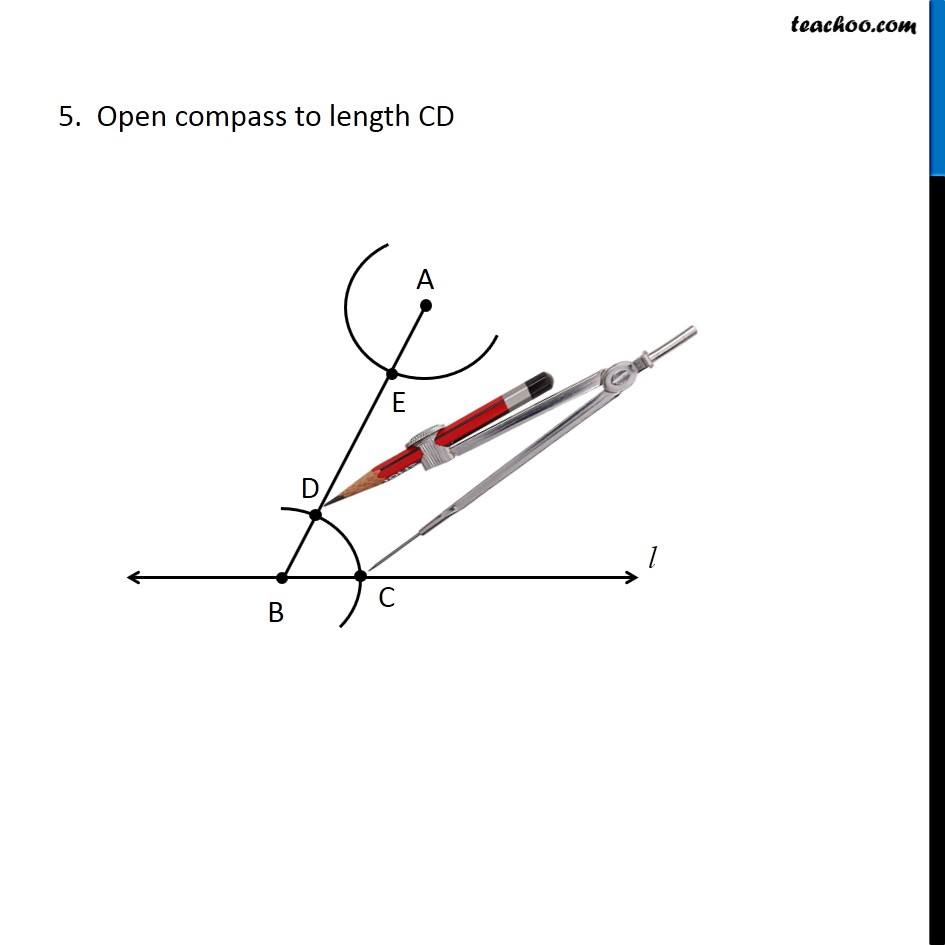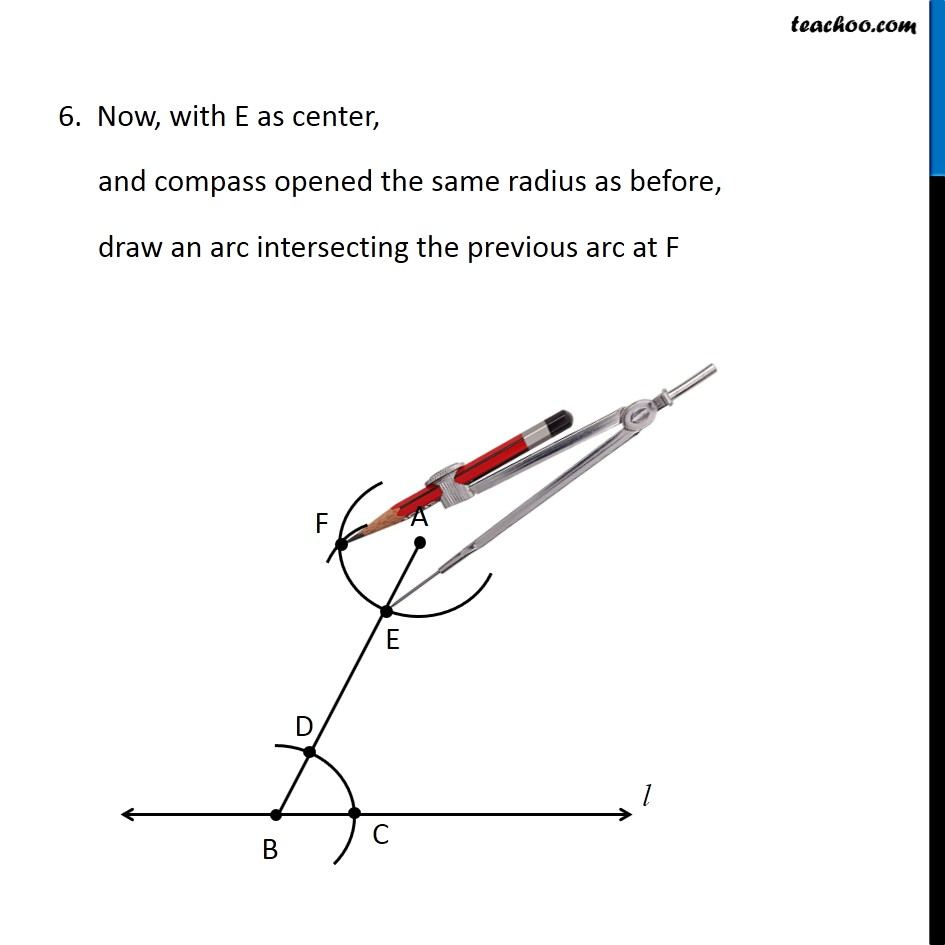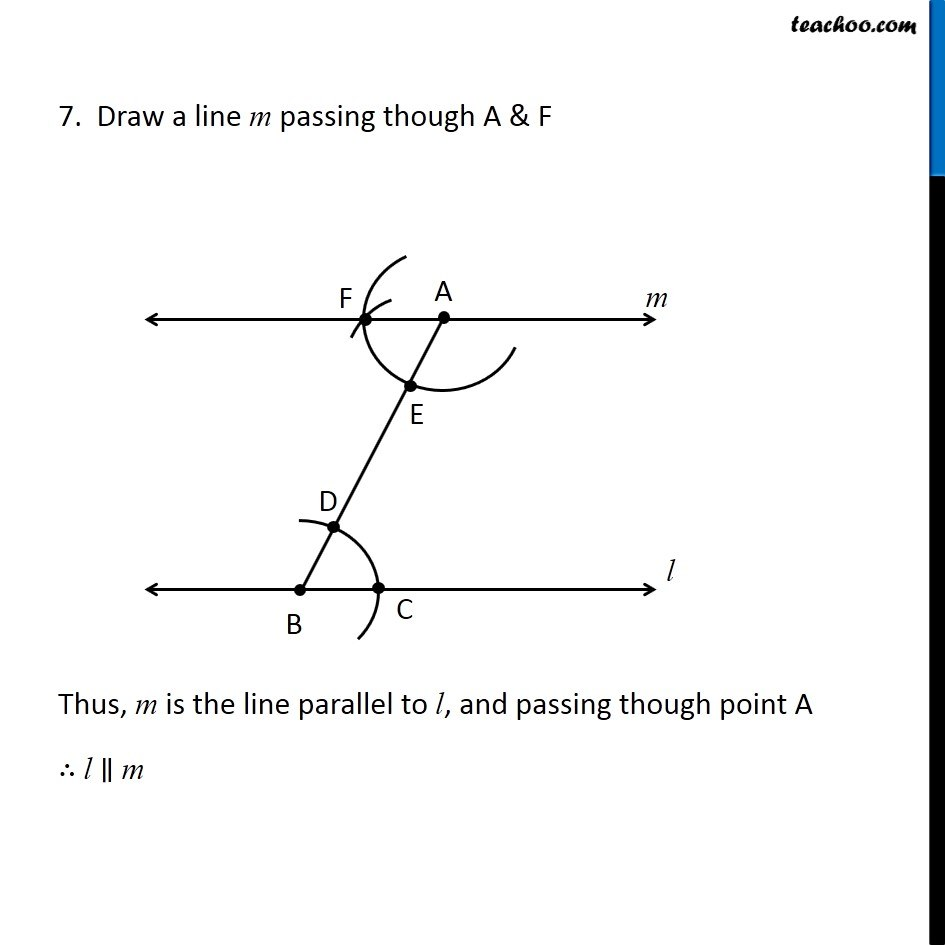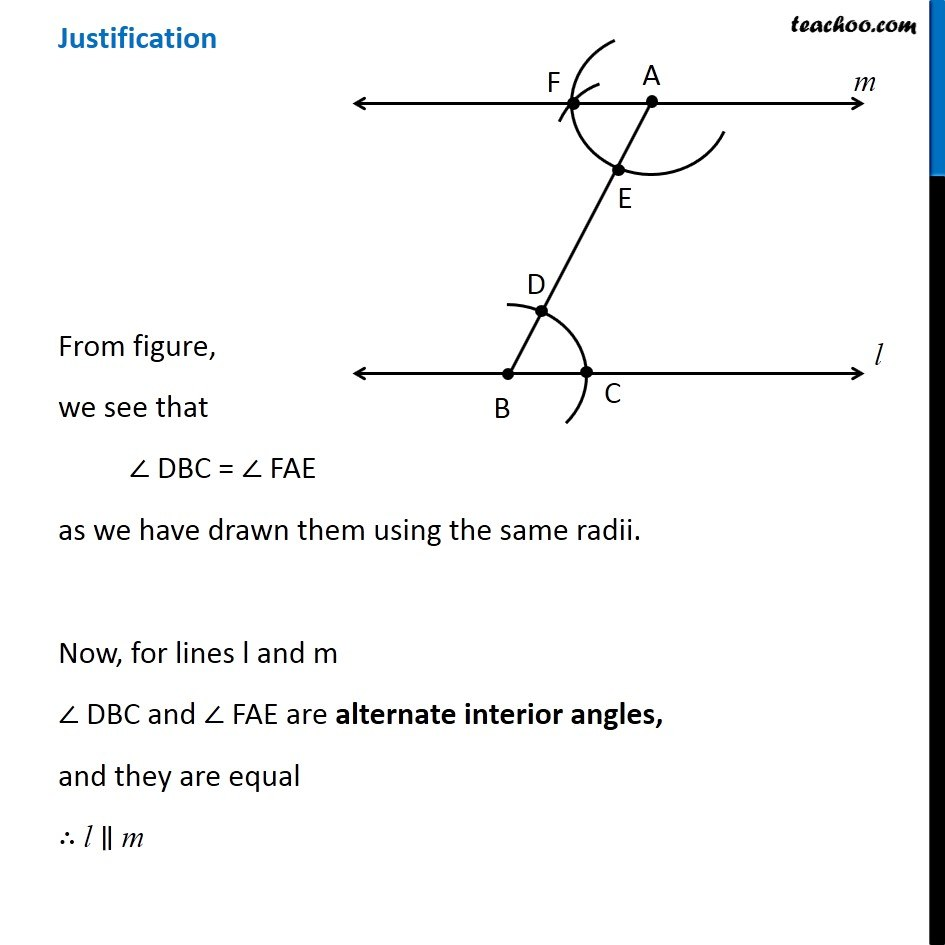Get live Maths 1-on-1 Classs - Class 6 to 12

### Transcript

Construction of a line parallel to a given line, through a point not on the line Let’s take an example 1. Given a line l with point A not on it We need to draw a line parallel to line l, passing through point A 2. Mark point B on the line l Join AB 3. With B as center, and any radius, draw an arc intersecting l at C, and AB at D 2. Mark point B on the line l Join AB 3. With B as center, and any radius, draw an arc intersecting l at C, and AB at D 5. Open compass to length CD 6. Now, with E as center, and compass opened the same radius as before, draw an arc intersecting the previous arc at F 7. Draw a line m passing though A & F Thus, m is the line parallel to l, and passing though point A ∴ l ∥ m Justification From figure, we see that ∠ DBC = ∠ FAE as we have drawn them using the same radii. Now, for lines l and m ∠ DBC and ∠ FAE are alternate interior angles, and they are equal ∴ l ∥ m